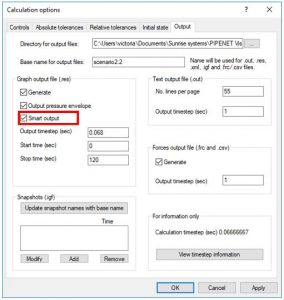### Smart Output: why it is important to choose this option

PIPENET Transient module users may sometimes notice discrepancies between the results shown in data and properties windows or generated reports, on the one hand, and the plotted graphs, on the other hand. Such discrepancies most probably arise due to mismatch between the calculation timestep and the output timestep. This issue can be easily solved by making sure that the Smart Output option is checked.PIPENET allows the user to select the calculation timestep and the output timestep. Naturally, the output timestep must be equal or larger than the calculation timestep. When the output timestep is larger than the calculated time step, the graph results may miss the peak values if they are located between output time interval. For example, the calculation time step is 0.01 sec and the graph output timestep is 0.05 sec. If a peak occurs at 24.53 sec, the graph only shows the results at 24.50 sec and 24.55 sec.

However, if the Smart Output option is selected, the graph will still “capture” all peak results even if the output timestep is larger than the calculation timestep.

Therefore, users are recommended to select the Smart Output option if the output timestep is larger than the calculation timestep, to avoid discrepancies in the results.